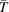International Tables for Crystallography (2006). Vol. C. ch. 6.3, pp. 599-608https://doi.org/10.1107/97809553602060000602

## Contents

• 6.3. X-ray absorption  (pp. 599-608)
• 6.3.1. Linear absorption coefficient  (pp. 599-600) | html | pdf |
• 6.3.1.1. True or photoelectric absorption  (p. 599) | html | pdf |
• 6.3.1.2. Scattering  (p. 599) | html | pdf |
• 6.3.1.3. Extinction  (pp. 599-600) | html | pdf |
• 6.3.1.4. Attenuation (mass absorption) coefficients  (p. 600) | html | pdf |
• 6.3.2. Dispersion  (p. 600) | html | pdf |
• 6.3.3. Absorption corrections  (pp. 600-608) | html | pdf |
• 6.3.3.1. Special cases  (p. 600) | html | pdf |
• 6.3.3.2. Cylinders and spheres  (pp. 600-604) | html | pdf |
• 6.3.3.3. Analytical method for crystals with regular faces  (pp. 604-606) | html | pdf |
• 6.3.3.4. Gaussian integration  (pp. 606-607) | html | pdf |
• 6.3.3.5. Empirical methods  (pp. 607-608) | html | pdf |
• 6.3.3.6. Measuring crystals for absorption  (p. 608) | html | pdf |
• References | html | pdf |
• Figures
• Fig. 6.3.1.1. Idealized diagram showing the variation of the photoelectric absorption coefficient σ ph with wavelength λ  (p. 599) | html | pdf |
• Fig. 6.3.3.1. Geometry of the Eulerian cradle with the axis of a cylindrical specimen coincident with the ϕ axis  (p. 604) | html | pdf |
• Fig. 6.3.3.2. Cross section of the plane of diffraction for a cylindrical specimen coincident with the ϕ axis  (p. 604) | html | pdf |
• Fig. 6.3.3.3. The crystal ABC divided into polygons by the dashed lines AE and CF parallel to the incident ( i ) and diffracted ( d ) beams, respectively  (p. 605) | html | pdf |
• Fig. 6.3.3.4. Crystal oriented with the normal N ( S ) to the face ABCD in the plane of view  (p. 608) | html | pdf |
• Fig. 6.3.3.5. Geometry of the Eulerian cradle in the bisecting position  (p. 608) | html | pdf |
• Tables
• Table 6.3.3.1. Transmission coefficients  (p. 601) | html | pdf |
• Table 6.3.3.2. Values of A * for cylinders  (p. 602) | html | pdf |
• Table 6.3.3.3. Values of A * for spheres  (p. 602) | html | pdf |
• Table 6.3.3.4. Values of (1/ A *)(d A */dμ R ) for spheres  (p. 603) | html | pdf |
• Table 6.3.3.5. Coefficients for interpolation of A * and(p. 603) | html | pdf |